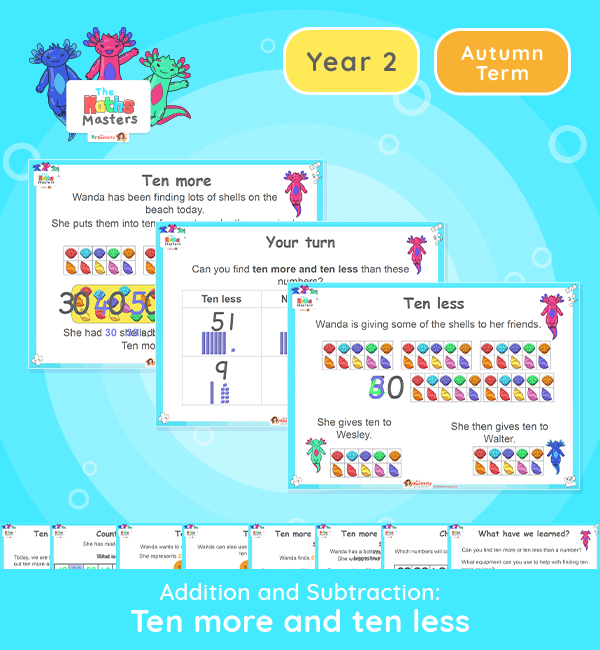# Year 2 | 10 More and 10 Less Lesson PresentationAligned with the maths mastery approach, this Year 2 | 10 More and 10 Less Lesson Presentation is fully editable, and is designed for the Year 2 maths curriculum covering the following maths objectives for the autumn term:

This lesson practises working out ten more or ten less than a number up to 100.

Small Steps: 10 more 10 less

NC Links: Recognise the place value of each digit in a two-digit number. Add and subtract numbers using concrete objects, pictorial representations and mentally, including a two-digit number and tens.

TAF Statements: Working Towards – Partition a two-digit numbers into tens and ones and demonstrate an understanding of place value.

Working At – Read scales in divisions of ones, twos, fives and tens.

Ready-to-progress criteria: Previous experience: Count forwards and backwards to and from 100.

2NPV-2 Reason about the location of any two-digit number in the linear number system, including identifying the previous and next multiple of 10.

2AS-3 Add and subtract within 100 by applying related one-digit addition adn subtract in facts: add and subtract only tens to/from a two-digit number.

Aligned with the order of teaching of the White Rose Maths scheme of work, use this to help your class get to grips with each mathematical concept. This lesson presentation also includes varied fluency activities, problem solving, and mathematical discussion questions too.

Explore our other  year 2 addition and subtraction resources.

## Recently Viewed